Inheritance

After death father (♱ 62) remained mother (his wife) and its 3 children. Inheritance by law is that in first mother will automatically get half of the property and other one half inherite by heirs witch are mother and her 3 children by same share.

Calculate the resulting share of property that falls to the mother and one child and express its as a percentage.

Result

M =  62.5 %
D =  12.5 %

Solution:Leave us a comment of example and its solution (i.e. if it is still somewhat unclear...):Be the first to comment!To solve this example are needed these knowledge from mathematics:

Need help calculate sum, simplify or multiply fractions? Try our fraction calculator. Our percentage calculator will help you quickly calculate various typical tasks with percentages.

Next similar examples:

1. Conference148 is the total number of employees. The conference was attended by 22 employees. How much is it in percent?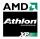I longer watch processors for Socket A on ebay, Athlon XP 1.86GHz with a PR rating of 2500+ costs \$7 and Athlon XP 2.16Ghz with a PR rating of 3000+ currently cost \$16. Calculate: About what percentage of the Athlon XP 2.16Ghz is powerful than Athlon X
3. VAT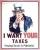VAT is a tax which the state artificially betrays goods and services for final consumption. VAT in Slovakia is 20%. Calculate how much percent pay less tax residents of Liechtenstein, when VAT is only 8%.
4. Typing course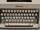Before taking a typing course, Terrence could type 39 words per minute. By the end of the course, he was able to type 68 words per minute. Find the percent increase.
5. Seeds 2How many seeds germinated from 1000 pcs, when 23% no emergence?
6. Profitability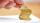The purchase price of goods is 13000, the sales price is the 20000. What is the profitability as a percentage?
7. Highway repairThe highway repair was planned for 15 days. However, it was reduced by 30%. How many days did the repair of the highway last?
8. TVsProduction of television sets increased from 3,500 units to 4,200 units. Calculate the percentage of production increase.
9. SaleA camera has a listed price of \$751.98 before tax. If the sales tax rate is 9.25%, find the total cost of the camera with sales tax included.
10. Percents - easyHow many percent is 432 out of 434?
11. IronIron ore contains 57% iron. How much ore is needed to produce 20 tons of iron?
12. ClassIn 7.C clss are 10 girls and 20 boys. Yesterday was missing 20% of girls and 50% boys. What percentage of students missing?
13. 100 %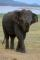How much is 100%, if 17 % is 1169?
14. Base, percents, valueBase is 344084 which is 100 %. How many percent is 384177?
15. Apples 2James has 13 apples. He has 30 percent more apples than Sam. How many apples has Sam?
16. Sales off 2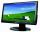Computer monitor sold for 8400 CZK in March. In April, dealer price decreased by 798 CZK. Calculate the percentage by which the price was reduced.
17. PercentsHow many percents is 900 greater than the number 750?